x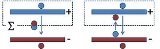CapacitanceOverview

In electromagnetism
Electromagnetism
Electromagnetism is one of the four fundamental interactions in nature. The other three are the strong interaction, the weak interaction and gravitation...

and electronics
Electronics
Electronics is the branch of science, engineering and technology that deals with electrical circuits involving active electrical components such as vacuum tubes, transistors, diodes and integrated circuits, and associated passive interconnection technologies...

, capacitance is the ability of a capacitor
Capacitor
A capacitor is a passive two-terminal electrical component used to store energy in an electric field. The forms of practical capacitors vary widely, but all contain at least two electrical conductors separated by a dielectric ; for example, one common construction consists of metal foils separated...

to store energy
Energy
In physics, energy is an indirectly observed quantity. It is often understood as the ability a physical system has to do work on other physical systems...

in an electric field
Electric field
In physics, an electric field surrounds electrically charged particles and time-varying magnetic fields. The electric field depicts the force exerted on other electrically charged objects by the electrically charged particle the field is surrounding...

. Capacitance is also a measure of the amount of electric potential energy
Electric potential energy
Electric potential energy, or electrostatic potential energy, is a potential energy that results from conservative Coulomb forces and is associated with the configuration of a particular set of point charges within a defined system...

stored (or separated) for a given electric potential
Electric potential
In classical electromagnetism, the electric potential at a point within a defined space is equal to the electric potential energy at that location divided by the charge there...

. A common form of energy storage device is a parallel-plate capacitor
Capacitor
A capacitor is a passive two-terminal electrical component used to store energy in an electric field. The forms of practical capacitors vary widely, but all contain at least two electrical conductors separated by a dielectric ; for example, one common construction consists of metal foils separated...

. In a parallel plate capacitor, capacitance is directly proportional to the surface area of the conductor plates and inversely proportional to the separation distance between the plates.Encyclopedia
In electromagnetism
Electromagnetism
Electromagnetism is one of the four fundamental interactions in nature. The other three are the strong interaction, the weak interaction and gravitation...

and electronics
Electronics
Electronics is the branch of science, engineering and technology that deals with electrical circuits involving active electrical components such as vacuum tubes, transistors, diodes and integrated circuits, and associated passive interconnection technologies...

, capacitance is the ability of a capacitor
Capacitor
A capacitor is a passive two-terminal electrical component used to store energy in an electric field. The forms of practical capacitors vary widely, but all contain at least two electrical conductors separated by a dielectric ; for example, one common construction consists of metal foils separated...

to store energy
Energy
In physics, energy is an indirectly observed quantity. It is often understood as the ability a physical system has to do work on other physical systems...

in an electric field
Electric field
In physics, an electric field surrounds electrically charged particles and time-varying magnetic fields. The electric field depicts the force exerted on other electrically charged objects by the electrically charged particle the field is surrounding...

. Capacitance is also a measure of the amount of electric potential energy
Electric potential energy
Electric potential energy, or electrostatic potential energy, is a potential energy that results from conservative Coulomb forces and is associated with the configuration of a particular set of point charges within a defined system...

stored (or separated) for a given electric potential
Electric potential
In classical electromagnetism, the electric potential at a point within a defined space is equal to the electric potential energy at that location divided by the charge there...

. A common form of energy storage device is a parallel-plate capacitor
Capacitor
A capacitor is a passive two-terminal electrical component used to store energy in an electric field. The forms of practical capacitors vary widely, but all contain at least two electrical conductors separated by a dielectric ; for example, one common construction consists of metal foils separated...

. In a parallel plate capacitor, capacitance is directly proportional to the surface area of the conductor plates and inversely proportional to the separation distance between the plates. If the charges on the plates are +q and −q, and V gives the voltage between the plates, then the capacitance is given by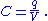The SI
Si
Si, si, or SI may refer to :- Measurement, mathematics and science :* International System of Units , the modern international standard version of the metric system...

unit of capacitance is the farad
The farad is the SI unit of capacitance. The unit is named after the English physicist Michael Faraday.- Definition :A farad is the charge in coulombs which a capacitor will accept for the potential across it to change 1 volt. A coulomb is 1 ampere second...

; 1 farad is 1 coulomb per volt
Volt
The volt is the SI derived unit for electric potential, electric potential difference, and electromotive force. The volt is named in honor of the Italian physicist Alessandro Volta , who invented the voltaic pile, possibly the first chemical battery.- Definition :A single volt is defined as the...

.

The energy
Energy
In physics, energy is an indirectly observed quantity. It is often understood as the ability a physical system has to do work on other physical systems...

(measured in joule
Joule
The joule ; symbol J) is a derived unit of energy or work in the International System of Units. It is equal to the energy expended in applying a force of one newton through a distance of one metre , or in passing an electric current of one ampere through a resistance of one ohm for one second...

s) stored in a capacitor is equal to the work done to charge it. Consider a capacitor of capacitance C, holding a charge +q on one plate and −q on the other. Moving a small element of charge dq from one plate to the other against the potential difference requires the work dW: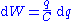where W is the work measured in joules, q is the charge measured in coulombs and C is the capacitance, measured in farads.

The energy stored in a capacitor is found by integrating
Integral
Integration is an important concept in mathematics and, together with its inverse, differentiation, is one of the two main operations in calculus...

this equation. Starting with an uncharged capacitance and moving charge from one plate to the other until the plates have charge +Q and −Q requires the work W: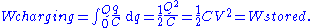## Capacitors

The capacitance of the majority of capacitors used in electronic circuits is several orders of magnitude smaller than the farad
The farad is the SI unit of capacitance. The unit is named after the English physicist Michael Faraday.- Definition :A farad is the charge in coulombs which a capacitor will accept for the potential across it to change 1 volt. A coulomb is 1 ampere second...

Femto-
Femto- is a prefix in the metric system denoting a factor of 10−15 or . Adopted by the 11th Conférence Générale des Poids et Mesures, it was added in 1964 to the SI...

Capacitance can be calculated if the geometry of the conductors and the dielectric properties of the insulator between the conductors are known. For example, the capacitance of a parallel-plate capacitor constructed of two parallel plates both of area A separated by a distance d is approximately equal to the following: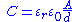where
C is the capacitance;
A is the area of overlap of the two plates;
εr is the relative static permittivity (sometimes called the dielectric constant) of the material between the plates (for a vacuum);
ε0 is the electric constant (ε0 ≈ ); and
d is the separation between the plates.

Capacitance is proportional to the area of overlap and inversely proportional to the separation between conducting sheets. The closer the sheets are to each other, the greater the capacitance.
The equation is a good approximation if d is small compared to the other dimensions of the plates so the field in the capacitor over most of its area is uniform, and the so-called fringing field around the periphery provides a small contribution. In CGS units
Gaussian units
Gaussian units comprise a metric system of physical units. This system is the most common of the several electromagnetic unit systems based on cgs units. It is also called the Gaussian unit system, Gaussian-cgs units, or often just cgs units...

the equation has the form: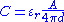where C in this case has the units of length.
Combining the SI equation for capacitance with the above equation for the energy stored in a capacitance, for a flat-plate capacitor the energy stored is: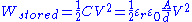.

where W is the energy, in joules; C is the capacitance, in farads; and V is the voltage, in volts.

### Voltage dependent capacitors

The dielectric constant for a number of very useful dielectrics changes as a function of the applied electrical field, for example ferroelectric materials, so the capacitance for these devices is more complex. For example, in charging such a capacitor the differential increase in voltage with charge is governed by: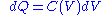where the voltage dependence of capacitance, C(V), stems from the field, which in a large area parallel plate device is given by ε = V/d. This field polarizes the dielectric, which polarization, in the case of a ferroelectric, is a nonlinear S-shaped function of field, which, in the case of a large area parallel plate device, translates into a capacitance that is a nonlinear function of the voltage causing the field.

Corresponding to the voltage-dependent capacitance, to charge the capacitor to voltage V an integral relation is found: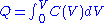which agrees with Q = CV only when C is voltage independent.

By the same token, the energy stored in the capacitor now is given by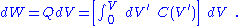Integrating: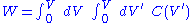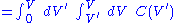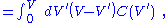where interchange of the order of integration
Order of integration (calculus)
In calculus, interchange of the order of integration is a methodology that transforms iterated integrals of functions into other, hopefully simpler, integrals by changing the order in which the integrations are performed...

is used.

The nonlinear capacitance of a microscope probe scanned along a ferroelectric surface is used to study the domain structure of ferroelectric materials.

Another example of voltage dependent capacitance occurs in semiconductor devices such as semiconductor diode
Diode
In electronics, a diode is a type of two-terminal electronic component with a nonlinear current–voltage characteristic. A semiconductor diode, the most common type today, is a crystalline piece of semiconductor material connected to two electrical terminals...

s, where the voltage dependence stems not from a change in dielectric constant but in a voltage dependence of the spacing between the charges on the two sides of the capacitor.

### Frequency dependent capacitors

If a capacitor is driven with a time-varying voltage that changes rapidly enough, then the polarization of the dielectric cannot follow the signal. As an example of the origin of this mechanism, the internal microscopic dipoles contributing to the dielectric constant cannot move instantly, and so as frequency of an applied alternating voltage increases, the dipole response is limited and the dielectric constant diminishes. A changing dielectric constant with frequency is referred to as dielectric dispersion, and is governed by dielectric relaxation processes, such as Debye relaxation. Under transient conditions, the displacement field can be expressed as (see electric susceptibility
Electric susceptibility
In electromagnetism, the electric susceptibility \chi_e is a dimensionless proportionality constant that indicates the degree of polarization of a dielectric material in response to an applied electric field...

):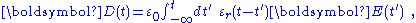indicating the lag in response by the time dependence of εr, calculated in principle from an underlying microscopic analysis, for example, of the dipole behavior in the dielectric. See, for example, linear response function
Linear response function
A linear response function describes the input-output relationship of a signal transducer such as a radio turning electromagnetic waves into music or a neuron turning synaptic input into a response...

. The integral extends over the entire past history up to the present time. A Fourier transform in time then results in: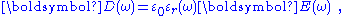where εr(ω) is now a complex function, with an imaginary part related to absorption of energy from the field by the medium. See permittivity. The capacitance, being proportional to the dielectric constant, also exhibits this frequency behavior. Fourier transforming Gauss's law with this form for displacement field: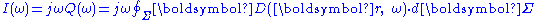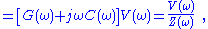where j is the imaginary unit
Imaginary unit
In mathematics, the imaginary unit allows the real number system ℝ to be extended to the complex number system ℂ, which in turn provides at least one root for every polynomial . The imaginary unit is denoted by , , or the Greek...

, V(ω) is the voltage component at angular frequency ω, G(ω) is the real part of the current, called the conductance, and C(ω) determines the imaginary part of the current and is the capacitance. Z(ω) is the complex impedance.

When a parallel-plate capacitor is filled with a dielectric, the measurement of dielectric properties of the medium is based upon the relation: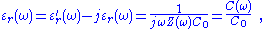where a single prime denotes the real part and a double prime the imaginary part, Z(ω) is the complex impedance with the dielectric present, C(ω) is the so-called complex capacitance with the dielectric present, and C0 is the capacitance without the dielectric. (Measurement "without the dielectric" in principle means measurement in free space, an unattainable goal inasmuch as even the quantum vacuum
Vacuum state
In quantum field theory, the vacuum state is the quantum state with the lowest possible energy. Generally, it contains no physical particles...

is predicted to exhibit nonideal behavior, such as dichroism
Dichroism
Dichroism has two related but distinct meanings in optics. A dichroic material is either one which causes visible light to be split up into distinct beams of different wavelengths , or one in which light rays having different polarizations are absorbed by different amounts.The original meaning of...

. For practical purposes, when measurement errors are taken into account, often a measurement in terrestrial vacuum, or simply a calculation of C0, is sufficiently accurate.)

Using this measurement method, the dielectric constant may exhibit a resonance
Resonance
In physics, resonance is the tendency of a system to oscillate at a greater amplitude at some frequencies than at others. These are known as the system's resonant frequencies...

at certain frequencies corresponding to characteristic response frequencies (excitation energies) of contributors to the dielectric constant. These resonances are the basis for a number of experimental techniques for detecting defects. The conductance method measures absorption as a function of frequency. Alternatively, the time response of the capacitance can be used directly, as in deep-level transient spectroscopy.

Another example of frequency dependent capacitance occurs with MOS capacitors, where the slow generation of minority carriers means that at high frequencies the capacitance measures only the majority carrier response, while at low frequencies both types of carrier respond.

At optical frequencies, in semiconductors the dielectric constant exhibits structure related to the band structure of the solid. Sophisticated modulation spectroscopy measurement methods based upon modulating the crystal structure by pressure or by other stresses and observing the related changes in absorption or reflection of light have advanced our knowledge of these materials.

## Capacitance matrix

The discussion above is limited to the case of two conducting plates, although of arbitrary size and shape. The definition C=Q/V still holds for a single plate given a charge, in which case the field lines produced by that charge terminate as if the plate were at the center of an oppositely charged sphere at infinity.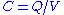does not apply when there are more than two charged plates, or when the net charge on the two plates is non-zero. To handle this case, Maxwell introduced his coefficients of potential. If three plates are given charges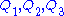, then the voltage of plate 1 is given by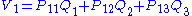,

and similarly for the other voltages. Hermann von Helmholtz
Hermann von Helmholtz
Hermann Ludwig Ferdinand von Helmholtz was a German physician and physicist who made significant contributions to several widely varied areas of modern science...

and Sir William Thomson showed that the coefficients of potential are symmetric, so that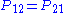, etc. Thus the system can be described by a collection of coefficients known as the elastance matrix or reciprocal capacitance matrix, which is defined as: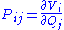From this, the mutual capacitance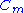between two objects can be defined by solving for the total charge Q and using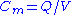.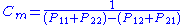Since no actual device holds perfectly equal and opposite charges on each of the two "plates", it is the mutual capacitance that is reported on capacitors.

The collection of coefficients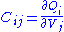is known as the capacitance matrix, and is the inverse of the elastance matrix.

## Self-capacitance

In electrical circuits, the term capacitance is usually a shorthand for the mutual capacitance
Mutual capacitance
Mutual capacitance is intentional or unintentional capacitance that occurs between two charge-holding objects or conductors, in which the current passing through one passes over into the other. Unlike mutual inductance, mutual capacitance only works along short distances...

between two adjacent conductors, such as the two plates of a capacitor. There also exists a property called self-capacitance, which is the amount of electrical charge that must be added to an isolated conductor to raise its electrical potential by one volt. The reference point for this potential is a theoretical hollow conducting sphere, of infinite radius, centered on the conductor. Using this method, the self-capacitance of a conducting sphere of radius R is given by: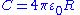Example values of self-capacitance are:
• for the top "plate" of a van de Graaff generator
Van de Graaff generator
A Van de Graaff generator is an electrostatic generator which uses a moving belt to accumulate very high voltages on a hollow metal globe on the top of the stand. It was invented in 1929 by American physicist Robert J. Van de Graaff. The potential differences achieved in modern Van de Graaff...

, typically a sphere 20 cm in radius: 20 pF
• the planet Earth
Earth
Earth is the third planet from the Sun, and the densest and fifth-largest of the eight planets in the Solar System. It is also the largest of the Solar System's four terrestrial planets...

The capacitative component of a coil, which reduces its impedance
Impedance
Impedance may refer to:*Electrical impedance, the ratio of the voltage phasor to the electric current phasor, a measure of the opposition to time-varying electric current in an electric circuit**Characteristic impedance of a transmission line...

at high frequencies and can lead to resonance
Resonance
In physics, resonance is the tendency of a system to oscillate at a greater amplitude at some frequencies than at others. These are known as the system's resonant frequencies...

and self-oscillation, is also called self-capacitance as well as stray or parasitic capacitance
Parasitic capacitance
In electrical circuits, parasitic capacitance, stray capacitance or, when relevant, self-capacitance , is an unavoidable and usually unwanted capacitance that exists between the parts of an electronic component or circuit simply because of their proximity to each other...

.

## Elastance

The reciprocal of capacitance is called elastance. The unit of elastance is the daraf
Daraf
The daraf is the unit of electrical elastance , the voltage across a capacitor after accepting an electric charge of 1 coulomb; it is the reciprocal of the farad...

, but is not recognised by SI.

## Stray capacitance

Any two adjacent conductors can be considered a capacitor, although the capacitance will be small unless the conductors are close together for long. This (often unwanted) effect is termed "stray capacitance". Stray capacitance can allow signals to leak between otherwise isolated circuits (an effect called crosstalk
Crosstalk (electronics)
In electronics, crosstalk is any phenomenon by which a signal transmitted on one circuit or channel of a transmission system creates an undesired effect in another circuit or channel...

), and it can be a limiting factor for proper functioning of circuits at high frequency
High frequency
High frequency radio frequencies are between 3 and 30 MHz. Also known as the decameter band or decameter wave as the wavelengths range from one to ten decameters . Frequencies immediately below HF are denoted Medium-frequency , and the next higher frequencies are known as Very high frequency...

.

Stray capacitance is often encountered in amplifier circuits in the form of "feedthrough" capacitance that interconnects the input and output nodes (both defined relative to a common ground). It is often convenient for analytical purposes to replace this capacitance with a combination of one input-to-ground capacitance and one output-to-ground capacitance. (The original configuration — including the input-to-output capacitance — is often referred to as a pi-configuration.) Miller's theorem can be used to effect this replacement. Miller's theorem states that, if the gain ratio of two nodes is 1/K, then an impedance
Electrical impedance
Electrical impedance, or simply impedance, is the measure of the opposition that an electrical circuit presents to the passage of a current when a voltage is applied. In quantitative terms, it is the complex ratio of the voltage to the current in an alternating current circuit...

of Z connecting the two nodes can be replaced with a Z/(1-k) impedance between the first node and ground and a KZ/(K-1) impedance between the second node and ground. (Since impedance varies inversely with capacitance, the internode capacitance, C, will be seen to have been replaced by a capacitance of KC from input to ground and a capacitance of (K-1)C/K from output to ground.) When the input-to-output gain is very large, the equivalent input-to-ground impedance is very small while the output-to-ground impedance is essentially equal to the original (input-to-output) impedance.

## Capacitance of simple systems

Calculating the capacitance of a system amounts to solving the Laplace equation ∇2φ=0 with a constant potential φ on the surface of the conductors. This is trivial in cases with high symmetry. There is no solution in terms of elementary functions in more complicated cases.

For quasi two-dimensional situations analytic functions may be used to map different geometries to each other. See also Schwarz-Christoffel mapping.
Capacitance of simple systems
Type Capacitance Comment
Parallel-plate capacitor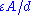A: Area
d: Distance
ε: Permittivity
Permittivity
In electromagnetism, absolute permittivity is the measure of the resistance that is encountered when forming an electric field in a medium. In other words, permittivity is a measure of how an electric field affects, and is affected by, a dielectric medium. The permittivity of a medium describes how...

Coaxial cable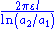a1: Inner radius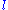: Length
Pair of parallel wires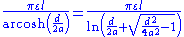a: Wire radius
d: Distance, d > 2a: Length of pair
Wire parallel to wall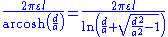a: Wire radius
d: Distance, d > a: Wire length
Two parallel
coplanar strips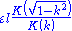d: Distance
w1, w2: Strip width
ki: d/(2wi+d)

k2: k1k2
K: Elliptic integral
Elliptic integral
In integral calculus, elliptic integrals originally arose in connection with the problem of giving the arc length of an ellipse. They were first studied by Giulio Fagnano and Leonhard Euler...: Length
Concentric spheres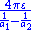a1: Inner radius
Two spheres,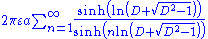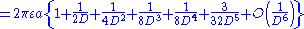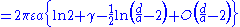d: Distance, d > 2a
D = d/2a
γ: Euler's constant
Euler-Mascheroni constant
The Euler–Mascheroni constant is a mathematical constant recurring in analysis and number theory, usually denoted by the lowercase Greek letter ....

Sphere in front of wall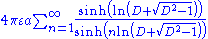a: Radius
d: Distance, d > a
D = d/a
Sphere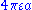a: Radius
Circular disc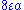a: Radius
Thin straight wire,
finite length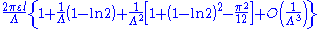a: Wire radius: Length
Λ: ln(/a)

• Ampère's law
Ampère's law
In classical electromagnetism, Ampère's circuital law, discovered by André-Marie Ampère in 1826, relates the integrated magnetic field around a closed loop to the electric current passing through the loop...

• Capacitor
Capacitor
A capacitor is a passive two-terminal electrical component used to store energy in an electric field. The forms of practical capacitors vary widely, but all contain at least two electrical conductors separated by a dielectric ; for example, one common construction consists of metal foils separated...

• Capacitive Displacement Sensors
• Conductance
Conductance
Conductance may refer to:* Electrical conductance, the ability for electricity to flow a certain path* Fluid conductance, the ability for fluid to transmit through materials* Thermal conductivity, the ability for temperatures to transmit through materials...

• Conductor
Electrical conductor
In physics and electrical engineering, a conductor is a material which contains movable electric charges. In metallic conductors such as copper or aluminum, the movable charged particles are electrons...

• Displacement current
Displacement current
In electromagnetism, displacement current is a quantity that is defined in terms of the rate of change of electric displacement field. Displacement current has the units of electric current density, and it has an associated magnetic field just as actual currents do. However it is not an electric...

• Electromagnetism
Electromagnetism
Electromagnetism is one of the four fundamental interactions in nature. The other three are the strong interaction, the weak interaction and gravitation...

• Electricity
Electricity
Electricity is a general term encompassing a variety of phenomena resulting from the presence and flow of electric charge. These include many easily recognizable phenomena, such as lightning, static electricity, and the flow of electrical current in an electrical wire...

• Electronics
Electronics
Electronics is the branch of science, engineering and technology that deals with electrical circuits involving active electrical components such as vacuum tubes, transistors, diodes and integrated circuits, and associated passive interconnection technologies...

• Hydraulic analogy
Hydraulic analogy
The electronic–hydraulic analogy is the most widely used analogy for "electron fluid" in a metal conductor. Since electric current is invisible and the processes at play in electronics are often difficult to demonstrate, the various electronic components are represented by hydraulic...

• Inductor
Inductor
An inductor is a passive two-terminal electrical component used to store energy in a magnetic field. An inductor's ability to store magnetic energy is measured by its inductance, in units of henries...

• Inductance
Inductance
In electromagnetism and electronics, inductance is the ability of an inductor to store energy in a magnetic field. Inductors generate an opposing voltage proportional to the rate of change in current in a circuit...

• Quantum capacitance
Quantum capacitance
Quantum capacitance is a physical value first introduced by Serge Luryi to describe the 2D-electronic systems in silicon surfaces and AsGa junctions. This capacitance was defined through standard density of states in the solids...